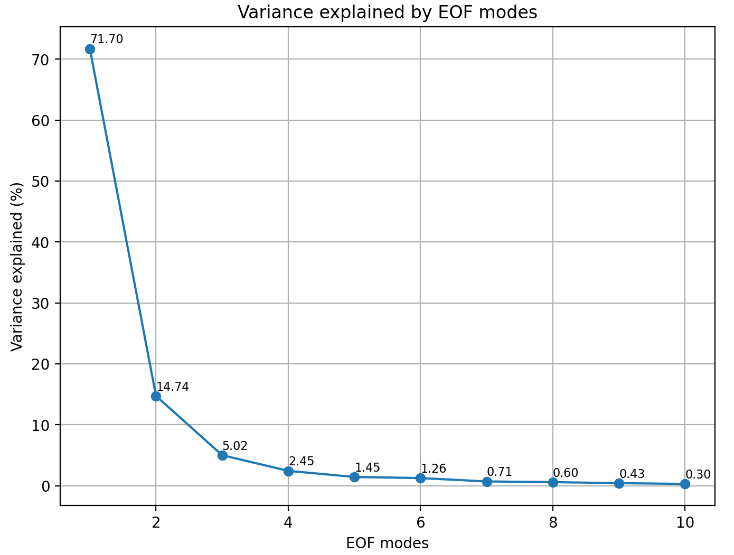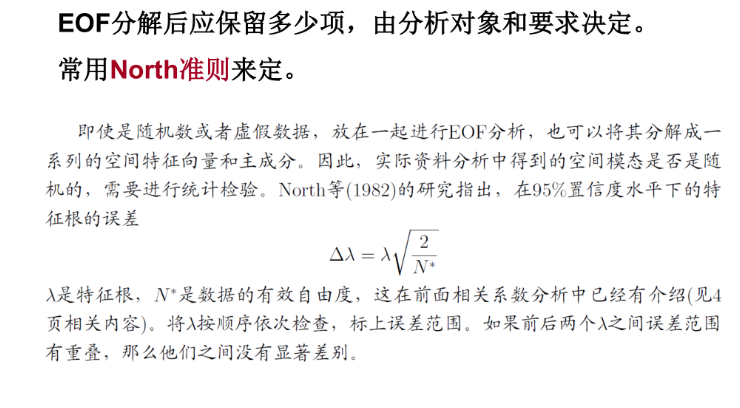V1

2023/05/12阅读：26主题：绿意

# 1.什么是经验正交分解

EOF分解是一种分析矩阵数据中的结构特征、提取主要数据特征量的方法。

EOF分解的分析对象，就是我们在气象科研和业务工作中，经常要分析各种气象要素场，如海表温度场、气压场、降水场等。这些气象要素场大多由不规则的网格点所组成。如果我们抽取这些场的某一段历史时期的资料，就构成一组以网格点为空间点(可看作多个变量)的随时间变化的样本。

EOF分解所做的，就是把随时间变化的气象要素场分解为 空间函数部分 和 时间函数部分，且这两部分相互正交。

# 3.在Python实现EOF分解

import structimport numpy as npimport xarray as xrfrom eofs.xarray import Eofimport matplotlib.pyplot as pltimport matplotlib.dates as mdatesimport matplotlib.ticker as tickerimport cartopy.crs as ccrs       import cartopy.feature as cfeature from cartopy.mpl.ticker import (LongitudeFormatter, LatitudeFormatter, LatitudeLocator, LongitudeLocator)import cmapsimport warningswarnings.filterwarnings('ignore')

## 3.1进行EOF分析

# 读取数据data = np.fromfile('ssta.dat', dtype='float32')data = np.reshape(data,(56, 11, 336), order='F')lon = np.arange(160, 272, 2) #经度160E~90W（间隔2度)lat = np.arange(-10, 12, 2)  #纬度10S~10N（间隔2度） time = xr.cftime_range(start='1979-01', end='2006-12', freq='MS') #时间1979.1~2006.12（逐月)ds = xr.Dataset({'ssta': ([ 'lon', 'lat', 'time'], data)},                coords={'lon': lon, 'lat': lat, 'time': time})#检验数据读取fig = plt.figure(figsize=(18, 12), dpi=300)  projection = ccrs.PlateCarree(central_longitude=180) #指定投影为经纬度投影，中心经纬度为180°ax1 = fig.add_subplot(1, 1, 1, projection=projection) #绘制第一个子图projection = ccrs.PlateCarree(central_longitude=180) #指定投影为经纬度投影，并指定中心经度为180°# 设置地图范围，经度为（160, 270)，纬度为（-10, 10）ax1.set_extent([-20, 90, -10, 10], crs=ccrs.PlateCarree(central_longitude=180))# 设置经纬度标签ax1.set_xticks([-20, -10, 0, 10, 20, 30, 40, 50, 60, 70, 80, 90], crs=projection)ax1.set_yticks([-10, -5, 0, 5, 10], crs=projection)lon_formatter = LongitudeFormatter(zero_direction_label=True) #给标签添加对应的N,S,E,Wlat_formatter = LatitudeFormatter()ax1.xaxis.set_major_formatter(lon_formatter)ax1.yaxis.set_major_formatter(lat_formatter)ax1.contourf(ds.lon,ds.lat,ds.ssta[:,:,0].transpose('lat', 'lon'), np.linspace(-1,1,51),cmap=cmaps.BlueWhiteOrangeRed,transform=ccrs.PlateCarree())  #在第一个地图上绘制第一空间模态的填充色图，EOFs表示从EOF中取出第一个空间模态ax1.coastlines(color='k', lw=0.5)                #添加海岸线ax1.add_feature(cfeature.LAND, facecolor='white') #添加陆地
# 转置数据ds = ds.transpose('time', 'lat', 'lon')#计算网格点的权重coslat = np.cos(np.deg2rad(ds.coords['lat'].values)) wgts = np.sqrt(coslat)[..., np.newaxis]# 计算EOF分解solver = Eof(ds['ssta'], weights=wgts, center=False)eofs = solver.eofs(neofs=10, eofscaling=2)pcs = solver.pcs(npcs=10, pcscaling=1)variance = solver.varianceFraction(neigs=10)# 绘制方差解释率图plt.figure(figsize=(8, 6),dpi=200)plt.plot(np.arange(1, 11), variance[:10]*100, 'o-')plt.xlabel('EOF modes')plt.ylabel('Variance explained (%)')plt.title('Variance explained by EOF modes')plt.grid()# 在每个点上添加文本标签for i, v in enumerate(variance.values[:10]*100):    plt.text(i+1, v+0.9, f'{v:.2f}', fontsize=8, color='black')plt.show()#计算特征值和NorthTest的结果eigenvalues = solver.eigenvalues(neigs=10)errors = solver.northTest(neigs=10)# 绘制Error Bar图plt.figure(figsize=(8, 6),dpi=200)plt.errorbar(np.arange(1, 11), eigenvalues, yerr=errors, fmt='o-', capsize=5)plt.xlabel('EOF modes')plt.ylabel('Eigenvalues')plt.title('NorthTest(with error bars)')plt.grid()plt.show()

## 3.2给出前4个模态的空间分布和时间序列（前4个modes的误差棒无相重叠部分）

import numpy as np
import matplotlib.pyplot as plt
import cartopy.crs as ccrs
import cartopy.feature as cfeature
from cartopy.mpl.gridliner import LongitudeFormatter, LatitudeFormatter
import cmaps

def mapart(ax):
'''
添加地图元素
'''
# 指定投影为经纬度投影，并指定中心经度为180°
projection = ccrs.PlateCarree(central_longitude=180)
# 设置地图范围，经度为（160, 270)，纬度为（-10, 10）
ax.set_extent([-20, 90, -10, 10], crs=ccrs.PlateCarree(central_longitude=180))
# 设置经纬度标签
ax.set_xticks([-20, -10, 0, 10, 20, 30, 40, 50, 60, 70, 80, 90], crs=projection)
ax.set_yticks([-10, -5, 0, 5, 10], crs=projection)
# 给标签添加对应的N,S,E,W
lon_formatter = LongitudeFormatter(zero_direction_label=True)
lat_formatter = LatitudeFormatter()
ax.xaxis.set_major_formatter(lon_formatter)
ax.yaxis.set_major_formatter(lat_formatter)
# 添加海岸线
#ax.coastlines(color='k', lw=0.5)
# 添加陆地

def eof_contourf(EOFs, PCs, pers):
'''
绘制EOF填充图
'''
plt.close
# 将方差转换为百分数的形式，如0.55变为55%
pers=(pers*100).values
# 指定画布大小以及像素
fig = plt.figure(figsize=(18, 12), dpi=300)
# 指定投影为经纬度投影，中心经纬度为180°
projection = ccrs.PlateCarree(central_longitude=180)
# 将横坐标转换为日期格式
dates_num = [mdates.date2num(t) for t in time]

# 绘制第一个子图
ax1 = fig.add_subplot(4, 2, 1, projection=projection)
# 为第一个子图添加地图底图
mapart(ax1)
# 在第一个地图上绘制第一空间模态的填充色图，EOFs表示从EOF中取出第一个空间模态
p = ax1.contourf(ds.lon,
ds.lat,
EOFs,
np.linspace(-1, 1, 51),
cmap=cmaps.BlueWhiteOrangeRed,
transform=ccrs.PlateCarree(),
extend='both')
# 为第一个子图添加标题，其中，pers表示从pers中取出第一个空间模态对应的方差贡献率
ax1.set_title('mode1 (%s' % (round(pers, 2))+"%)", loc='left')
ax1.set_aspect(1.6)

# 绘制第二个子图
ax2.set_xticks(dates_num[::108])  # 每9年的1月作为横坐标标签
ax2.xaxis.set_major_formatter(mdates.DateFormatter('%Y-%m'))
ax2.xaxis.set_major_locator(ticker.FixedLocator(dates_num[::108]))  # 每9年的1月作为横坐标刻度线
b = ax2.bar(dates_num, PCs[:, 0], width=50, color='r')
# 对时间系数值小于0的柱子设置为蓝色
for bar, height in zip(b, PCs[:, 0]):
if height < 0:
bar.set(color='blue')
# 为第二个子图添加标题
ax2.set_title('PC1' % (round(pers, 2)), loc='left')

# 后面ax3和ax5与ax1绘制方法相同，ax4和ax6与ax2绘制方法相同，不再注释

ax3 = fig.add_subplot(4, 2, 3, projection=projection)
mapart(ax3)
pp = ax3.contourf(ds.lon,
ds.lat,
EOFs,
np.linspace(-1, 1, 51),
cmap=cmaps.BlueWhiteOrangeRed,
transform=ccrs.PlateCarree(),
extend='both')
ax3.set_title('mode2 (%s' % (round(pers, 2))+"%)", loc='left')
ax3.set_aspect(1.6)

ax4.set_xticks(dates_num[::108])  # 每9年的1月作为横坐标标签
ax4.xaxis.set_major_formatter(mdates.DateFormatter('%Y-%m'))
ax4.xaxis.set_major_locator(ticker.FixedLocator(dates_num[::108]))  # 每9年的1月作为横坐标刻度线
ax4.set_title('PC2' % (round(pers, 2)), loc='left')
bb = ax4.bar(dates_num, PCs[:, 1], width=50, color='r')
for bar, height in zip(bb, PCs[:, 1]):
if height < 0:
bar.set(color='blue')

ax5 = fig.add_subplot(4, 2, 5, projection=projection)
mapart(ax5)
ppp = ax5.contourf(ds.lon,
ds.lat,
EOFs,
np.linspace(-1, 1, 51),
cmap=cmaps.BlueWhiteOrangeRed,
transform=ccrs.PlateCarree(),
extend='both')
ax5.set_title('mode3 (%s' % (round(pers, 2))+"%)", loc='left')
ax5.set_aspect(1.6)

ax6.set_xticks(dates_num[::108])  # 每9年的1月作为横坐标标签
ax6.xaxis.set_major_formatter(mdates.DateFormatter('%Y-%m'))
ax6.xaxis.set_major_locator(ticker.FixedLocator(dates_num[::108]))  # 每9年的1月作为横坐标刻度线
ax6.set_title('PC3' % (round(pers, 2)), loc='left')
bbb = ax6.bar(dates_num, PCs[:, 2], width=50, color='r')
for bar, height in zip(bbb, PCs[:, 2]):
if height < 0:
bar.set(color='blue')

ax7 = fig.add_subplot(4, 2, 7, projection=projection)
mapart(ax7)
pppp = ax7.contourf(ds.lon,
ds.lat,
EOFs,
np.linspace(-1, 1, 51),
cmap=cmaps.BlueWhiteOrangeRed,
transform=ccrs.PlateCarree(),
extend='both')
ax7.set_title('mode4 (%s' % (round(pers, 2))+"%)", loc='left')
ax7.set_aspect(1.6)

ax8.set_xticks(dates_num[::108])  # 每9年的1月作为横坐标标签
ax8.xaxis.set_major_formatter(mdates.DateFormatter('%Y-%m'))
ax8.xaxis.set_major_locator(ticker.FixedLocator(dates_num[::108]))  # 每9年的1月作为横坐标刻度线
#ax8.set_xlim(dates_num, dates_num[-1])
bbbb = ax8.bar(dates_num, PCs[:, 3], width=50, color='r')
for bar, height in zip(bbbb, PCs[:, 3]):
if height < 0:
bar.set(color='blue')
ax8.set_title('PC4' % (round(pers, 2)), loc='left')

# 为柱状图添加0标准线
ax2.axhline(y=0,  linewidth=1, color='k', linestyle='-')
ax4.axhline(y=0,  linewidth=1, color='k', linestyle='-')
ax6.axhline(y=0,  linewidth=1, color='k', linestyle='-')
ax8.axhline(y=0,  linewidth=1, color='k', linestyle='-')

# 在图下边留白边放colorbar
# colorbar位置： 左 下 宽 高
l = 0.25
b = 0.04
w = 0.6
h = 0.015
# 对应 l,b,w,h；设置colorbar位置；
rect = [l, b, w, h]

# 绘制colorbar
c = plt.colorbar(
p,
cax=cbar_ax,
orientation='horizontal',
aspect=20,

# 设置colorbar的标签大小
c.ax.tick_params(labelsize=14)

# 调整子图之间的间距
plt.show()

eof_contourf(eofs[:4]*(-1),pcs[:,:4]*(-1),variance[:4])


《气象统计分析与预报方法第4版 黄嘉佑》

USTC气象统计方法课程-郑建秋

### 参考资料





《气象统计分析与预报方法第4版 黄嘉佑》: as



USTC气象统计方法课程-郑建秋: as



Python

V1